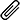# Answering to All Assessments Questions

High Quality, Fast Delivery, Plagiarism Free - Just in 3 Steps

## Submit Your Question HereMultiple Attach File

# Financial Statement Analysis -Fundamental Analysis and the Information Required

Each section will require approximately 500 words (approximately 2,000 words in total) – Obviously more words for questions that require explanations than calculations. Please also consider the marks for each question, which are identified at the end of the question.
Questions are as follows;
Question 1 – 25 marks
a) As at 1 July 2015, a T-bond had the listed spot price being \$95.40, and the listed futures price being \$95.60. An investor sold \$100,000 T-bonds at par value, and bought a T-bond futures contract. On 1 August 2015, the T-bond’s listed spot price was \$95.00, and listed futures price was \$94.40. Calculate the profit should the investor decide to liquidate his position. (5 marks).
b) Identify and explain five differences between futures and forward contracts. (20 marks).
Question 2 – 25 marks
Equity valuation is based purely on accounting information on the firm’s performance. It does not require the analyst to access information outside the company’s financial statements in order to forecast company earnings and returns.
a) Provide a critical but brief evaluation of this statement based on your understanding of the role of fundamental analysis in determining equity value. (13 marks).
b) Consider the process of equity valuation based on fundamental analysis and the information required for this process. What types of information would prove useful to the equity analyst? From where would each form of information that you mention be sourced? (12 marks).
Question 3 – 25 marks
An investor is evaluating three investment funds, for which the average returns, standard deviations and betas are given below. Assume the risk-free return during the relevant period is 8%.
 Average Return Standard Deviation Beta Fund 1 22% 25% 1.3 Fund 2 20% 18% 1.2 Fund 3 15% 12% 1.1
a) Distinguish Sharpe’s measure from Treynor’s measure. (13 marks).
b) Fir each of the above 3 funds, determine: i) the Sharpe measure, ii) the Treynor measure. (12 marks).
Question 4 – 25 marks
a) Identify three variables that determine option prices, and discuss the relationship among these variables. Your answer should distinguish, where relevant, between put and call options. (15 marks).
b) The follow data relates to a call option:
SO= \$70; X = \$70; T = 0.2 years; r = 0.06 annually; σ = 0.4 (annually).
No dividends will be paid before the option expires. Determine the value of the call option using the Black-Scholes Option Pricing Model. (10 marks).
Be Continue….

### Accounting Case Studies Assignment

At Casestudyhelp.com, our motive is to impart knowledge in the field of accounting, help the students to thoroughly understanding the subject and get good grades with our accounting writing Case Study Analysis for MBA.

#### Get This Answer with Case Study Help-24/7 Q&A Help

We're here to instantly help! Get best assignment questions and answers help 24/7 and Earn better grades with homework

PLACE YOUR ORDER HERE

Top